## RS Aggarwal Class 9 Solutions Chapter 2 Polynomials Ex 2J

These Solutions are part of RS Aggarwal Solutions Class 9. Here we have given RS Aggarwal Solutions Class 9 Chapter 2 Polynomials Ex 2J.

Other Exercises

Factorize :

Question 1.
Solution:
x3 + 27
= (x)3 + (3)3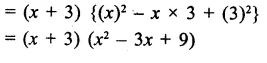Question 2.
Solution:
8x3 + 27y3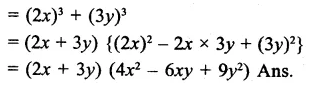Question 3.
Solution:
343 + 125b3Question 4.
Solution:
(1)3+(4x)3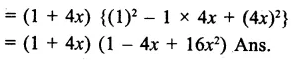Question 5.
Solution:
125a3+ $$\frac { 1 }{ 8 }$$Question 6.
Solution:
216x3+$$\frac { 1 }{ 125 }$$Question 7.
Solution:
16x4 + 54xQuestion 8.
Solution:
7a3 + 56b3
=7(a3+8b3)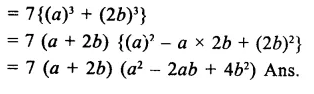Question 9.
Solution:
x5 + x2
=x2(x3+1)Question 10.
Solution:
a3 + 0.008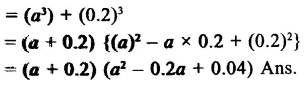Question 11.
Solution:
x6 + y6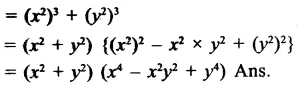Question 12.
Solution:
2a3 + 16b3 – 5a – 10bQuestion 13.
Solution:
x3 – 512
=(x)– (8)3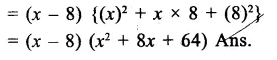Question 14.
Solution:
64x3 – 343
=(4x)– (7)3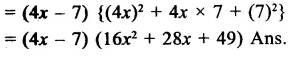Question 15.
Solution:
1 – 27x3
=(1)3– (3x)3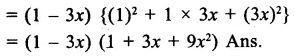Question 16.
Solution:
x3 – 125y3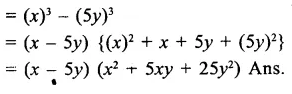Question 17.
Solution:
8x3 – $$\frac { 1 }{ { 27y }^{ 3 } }$$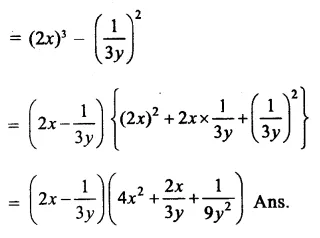Question 18.
Solution:
a3 – 0.064
=(a)– (0.4)3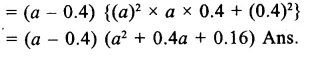Question 19.
Solution:
(a + 6)3 – 8
=(a+b)– (2)3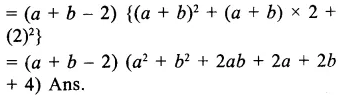Question 20.
Solution:
x6 – 729
=(x2)– (9)3Question 21.
Solution:
(a + b)3 – (a – b)3Question 22.
Solution:
x – 8xy3
=x(1 – 8y3)
=x{(1)– (2y)3}Question 23.
Solution:
32x4 – 500xQuestion 24.
Solution:
3a7b – 81a4 b4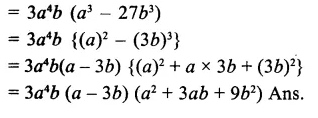Question 25.
Solution:
$${ a }^{ 3 }-\frac { 1 }{ { a }^{ 3 } } -2a+\frac { 2 }{ a }$$Question 26.
Solution:
8a3 – b3 – 4ax + 2bxQuestion 27.
Solution:
a3 + 3a2b + 3ab2 + b3 – 8Hope given RS Aggarwal Solutions Class 9 Chapter 2 Polynomials Ex 2J are helpful to complete your math homework.

If you have any doubts, please comment below. Learn Insta try to provide online math tutoring for you.